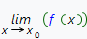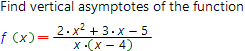# Vertical asymptotes online calculatorVertical asymptote of the function f(x) called the straight line parallel y axis that is closely appoached by a plane curve f(x). The distance between this straight line and the plane curve tends to zero as x tends to the infinity. The vertical asymptote equation has the form:

x = x0, where x0 - some constant (finity number)

The vertical asymptote of the function f(x) exists if the value of both (or one of the) limitsandequals to +∞ or −∞.

It should be noted that the limits described above also used to test whether the point x = x0 is the discontinuity point of the function f(x). Hence, the vertical asymptotes should only be searched at the discontinuity points of the function.

Use our online calculator, based on the Wolfram Aplha system, to find vertical asymptotes of your function.

Vertical asymptotes calculatorFunction which vertical asymptotes you want to find: